# Mixed numbers + adding - math problems

#### Number of problems found: 64

• Nick was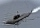Nick was scuba diving at -32 1/2 feet. If he descends another 8 3/5 feet, what is his location?
• A land 2A land owner owns two parcels of land measuring 4 2/5 hectares and 6 1/5 hectares. He sells 3/4 of the total land. How many hectares of the land are left?
• AlishaAlisha needs 7 1/2 yards of fabric to make a quilt. She has one piece of fabric that is 1 1/2 yards and another piece of fabric that is 3 1/4 yards. How many more yards of fabric does she need to make a quilt?
• Zailene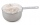Zailene wants to make two kinds of kakanin, puto and kutsina. Puto recipe needs 3 2/5 cup of flour while Kutsinta needs 5 ¼ cup of flour. How many cups of flour will Zailene be needed in all?
• A more than bWhat is 5 1/7 more than 2 3/7?
• Simplify expression with mixedWhen (7¹/3 + 2¾)÷(2 + 2¼ × 1¹/3) is simplified the result is?
• Evaluate 17Evaluate 2x+6y when x=- 4/5 and y=1/3. Write your answer as a fraction or mixed number in simplest form.Add this two mixed numbers: 1 5/6 + 2 2/11=
• Evaluate fractionsThe difference  of 5 1/2 and 2/3 is added to the product of 5/6 and 1/2
• A science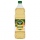A science experiment calls for making 3 and 2/3 cups of distilled water with 1 and 3/5 cups of vinegar and 2/3 cups of liquid detergent how much liquid in all and cups is needed?
• Sum of 20Sum of the two numbers is 15 1/6. If one of the numbers is 2 2/3, find the other number.
• The sum 12The sum of 3 mixed numbers is 20 13/15. two of the numbers are 6 1/3 and 7 5/6. what is the third number?
• Pen and pencil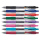Cost of a pen is Rs. 12 3/5 and cost of a pencil is Rs. 3 2/5. What is the total cost of pen and pencil?
• A full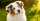A full bag of dog food weights 5 1/2 pounds. How much dog food do you have if there are 3 3/4 bags in the cupboard?
• BookshelfBill is cutting boards to build a bookshelf for his apartment. While cutting boards he accidently cut 3/16th ft. His board is 3 3/4th, how long should it have been?
• Packets weight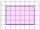The weight of three packets are 3 2/5 kg + 2 1/3 kg + 5 1/2 kg. Find total weight of all the three packets.
• Sum of mixedThe sum of two mixed numbers is 15½. One of the number is 5 ¾, what is the other?
• Sum of 18Sum of two fractions is 4 3/7. If one of the fractions is 2 1/5 find the other one .
• A man 3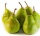A man buys a box of fruits containing 286 fruits out of these 1/2 are apples and the rest are pears. 4/13 of the pears are rotten. He sells the good pears at rupees 4 1/11 each. How much money does he receive on selling the good pears?
• Donuts 3You pick up donuts for a party. You have 3 and 4/12 boxes of donuts. Roy picks up donuts too. He has 2 and 9/12 boxes of donuts. How many boxes of donuts do you have in all?

Do you have an exciting math question or word problem that you can't solve? Ask a question or post a math problem, and we can try to solve it.

We will send a solution to your e-mail address. Solved examples are also published here. Please enter the e-mail correctly and check whether you don't have a full mailbox.

Please do not submit problems from current active competitions such as Mathematical Olympiad, correspondence seminars etc...

Mixed numbers - math problems. Adding Problems.